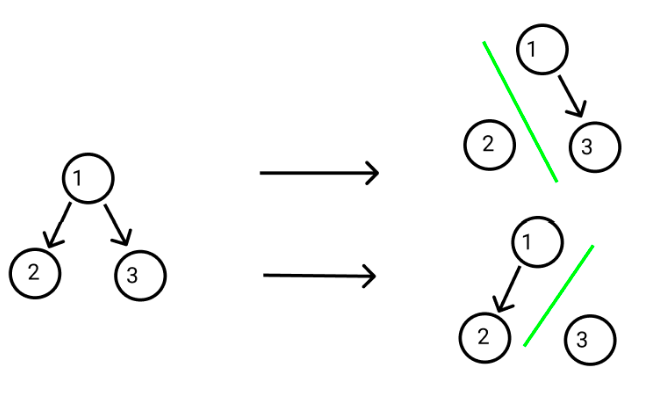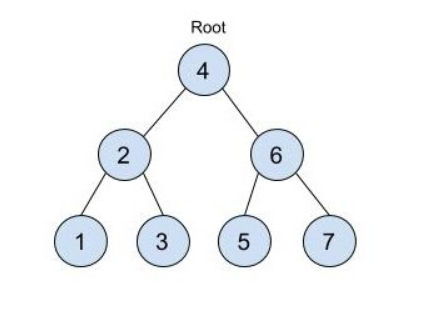# Equal Tree Partition

Posted: 24 Mar, 2021
Difficulty: Moderate

## PROBLEM STATEMENT

#### For example:``````In this given ‘BINARY_TREE’, we can make two possible partitions:
1. The first one is with sums 2 and 4.
2. The second one is with sums 3 and 3.

The second partition divides the given tree into an equal sum partition tree, so we return “True”.
``````
##### Input Format
``````The first line of input contains an integer 'T' representing the number of test cases. Then the test cases follow.

The next line of each test case contains elements in the level order form. The line consists of values of nodes separated by a single space. In case a node is null, we take -1 in its place.

The next line of each test case contains one integer ‘K’ representing that the houses that are still not vaccinated are at a ‘K’ distance from the last houses in the city.

For example, the input for the tree depicted in the below image would be :
````````````4
2 6
1 3 5 7
-1 -1 -1 -1 -1 -1
``````

#### Explanation :

``````Level 1 :
The root node of the tree is 4

Level 2 :
Left child of 4 = 2
Right child of 4 = 6

Level 3 :
Left child of 2 = 1
Right child of 2 = 3
Left child of 6 = 5
Right child of 6 = 7

Level 4 :
Left child of 1 = null (-1)
Right child of 1 = null (-1)
Left child of 3 = null (-1)
Right child of 3 = null (-1)
Left child of 5 = null (-1)
Right child of 5 = null (-1)
Left child of 7 = null (-1)
Right child of 7 = null (-1)

The first not-null node(of the previous level) is treated as the parent of the first two nodes of the current level. The second not-null node (of the previous level) is treated as the parent node for the next two nodes of the current level and so on.

The input ends when all nodes at the last level are null(-1).
``````
##### Note :
``````The above format was just to provide clarity on how the input is formed for a given tree.

The sequence will be put together in a single line separated by a single space. Hence, for the above-depicted tree, the input will be given as:

4 2 6 1 3 5 7 -1 -1 -1 -1 -1 -1
``````
##### Output Format :
``````For each test case, return “True” if we can partition the given ‘BINARY_TREE’ into two trees with equal sum values else return “False”.

Print the output of each test case in a separate line.
``````

#### Note: You do not need to print anything; it has already been taken care of. Just implement the given function.

##### Constraints :
``````1 <= ‘T’ <= 10
1 <= ‘DATA’ <= 10^5

Time Limit: 1 second
``````Approach 1

As we know we can remove exactly on edge from the given binary tree. So first, we calculate the total sum of all nodes of the ‘BINARY_TREE’. If the sum is odd, that means we cannot divide the ‘BINARY_TREE’ into two trees with equal sum values, so we return “false”. Else we traverse the tree and at every node, we check if the sum of the subtree is equal to the total sum of all nodes by 2. If it is true, then we return true else we repeat this for another subtree.

Here is the algorithm:

1. First, we traverse the tree and find the total sum of all nodes of the ‘BINARY_TREE’ and store it into ‘TOTAL_SUM’.
2. If ‘TOTAL_SUM’ is odd:
1. Return false.
3. We traverse the whole ‘BINARY_TREE’ and at every node, we calculate the sum of nodes of the left subtree and the right subtree.
4. ‘CURR_SUM’ = sumOfSubTreeHelper(root).
5. If the ‘CURR_SUM’ is equal to ‘TOTAL_SUM’ by 2:
1. Return true.
6. Finally, we return false.

sumOfSubTreeHelper(‘ROOT’)

1. If ‘ROOT’ is equal to ‘NULL’:
1. Return 0.
2. ‘TOTAL_SUM’ = ‘ROOT’.data +  sumOfSubTreeHelper(‘ROOT’.left) + sumOfSubTreeHelper(‘ROOT’.right).
3. Finally, return ‘TOTAL_SUM’.